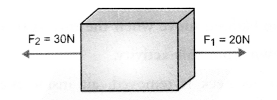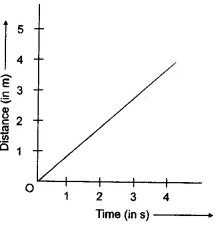## HOTS Questions for Class 9 Science Chapter 9 Force and Laws of Motion

These Solutions are part of HOTS Questions for Class 9 Science. Here we have given HOTS Questions for Class 9 Science Chapter 9 Force and Laws of Motion

Question 1.
The force of friction between the surface of a floor and the surface of a box in contact with the floor in 200 N. We wish to move the box on the floor with constant velocity. How much force has to be applied on the box ?
The box will move with a constant velocity if no net external force acts on the body. Thus, the effect of force of friction has to be balanced. So a force equal to the force of friction (i.e., 200 N) but opposite in direction has to be applied on the box to move it with a constant velocity. So the applied force on the box = 200 N.

More Resources

Question 2.
A force of 100 N acts on a body moving with a constant velocity of 20 m s-1 on a floor in a straight line. What is the force of friction between the body and the floor ?
Since, body is moving with a constant velocity, so no net force acts on the body. Hence, the force of 100 N acting on the body must be balanced by the force of friction between the body and the floor in contact. Therefore, force of friction between the body and the floor = 100 N and acts in the direction opposite to the direction of force acting on the body.

Question 3.
Two forces F1 = 20 N and F2 = 30 N are acting on an object as shown in figure.1. What is the net force acting on the object ?
2. What is the direction of the net force acting on the object ?
3. How much extra force is acting on the object if the object is not moving due to the application of these two forces ? Name that force. Where that force acts and what is the direction of that force ? (CBSE 2011, 2013)

1. Net force acting on the object = F2 – F1 = 30 N – 20 N = 10 N
2. Net force acts in the direction of the force F2.
3. Since the object is not moving, so net external force acting on it is zero. If extra force = F3, then
F1 + F2 + F3 = 0    or
20 N – 30 N + F3 = 0
(Direction of F1 is taken as +ve and direction of F2 is taken as -ve). or -10 N + F3 =0
or F3 = 10 N
This force F3 is known as force of friction. Force of friction acts between the lower surface of the object and the upper surface of the floor. Direction of force of friction is same as that of the direction of force F1.

Question 4.
Two identical bullets are fired, one by a light rifle and another by a heavy rifle with the same force. Which rifle will hurt the shoulder more and why ? (NCERT Question Bank)

Or

Why does the recoil of a heavy gun on firing not so strong as of a light gun using the same cartridges ?
(CBSE 2011, 2012)